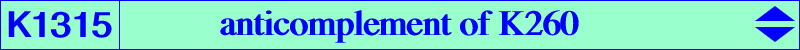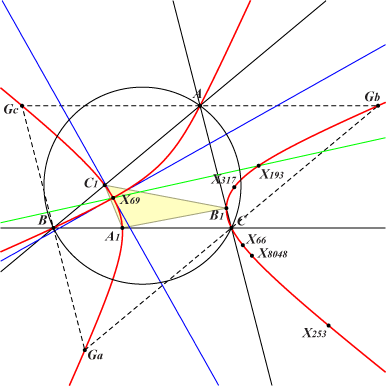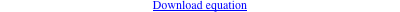too complicated to be written here. Click on the link to download a text file.X(66), X(69), X(193), X(253), X(317), X(8048), X(40697) isogonal conjugate of X(3162) anticomplements of X(219) and X(14390) vertices of the antimedial triangle with tangents concurring at X(11442) = anticomplement of X(184) infinite points of K1314 and all the pKs mentioned there Geometric properties :K1315 is a cubic related to K1314. These two cubics share the same infinite points and meet again at six points on the circum-conic with center and perspector the barycentric products X(193) × X(115) and X(193) × X(525) respectively. These points are A, B, C, X(193) and X(69) twice. K1315 is a nodal cubic with node X(69) and nodal tangents parallel to the asymptotes of the Jerabek hyperbola. K1315 is the anticomplement of K260, the isotomic transform of K617, the isotomic transform of the anticomplement K009, the barycentric product X(6) × K389. K1315 meets the sidelines of ABC at the vertices of A1B1C1, perspective to the antipedal triangle of X(24). The perspector is a^2 (a^4-2 a^2 b^2+b^4+2 b^2 c^2-c^4) (a^4+b^4-2 b^2 c^2+c^4) (a^4-b^4-2 a^2 c^2+2 b^2 c^2+c^4) : : , SEARCH = 0.148173274711096, on the line {157,523}.